Lesson 21. UNITS. EARTH

• The purpose of this lesson

Hi! Today we will get acquainted with the earth blocks and learn how to determine the soil moisture in a pot with a plant (figure 1).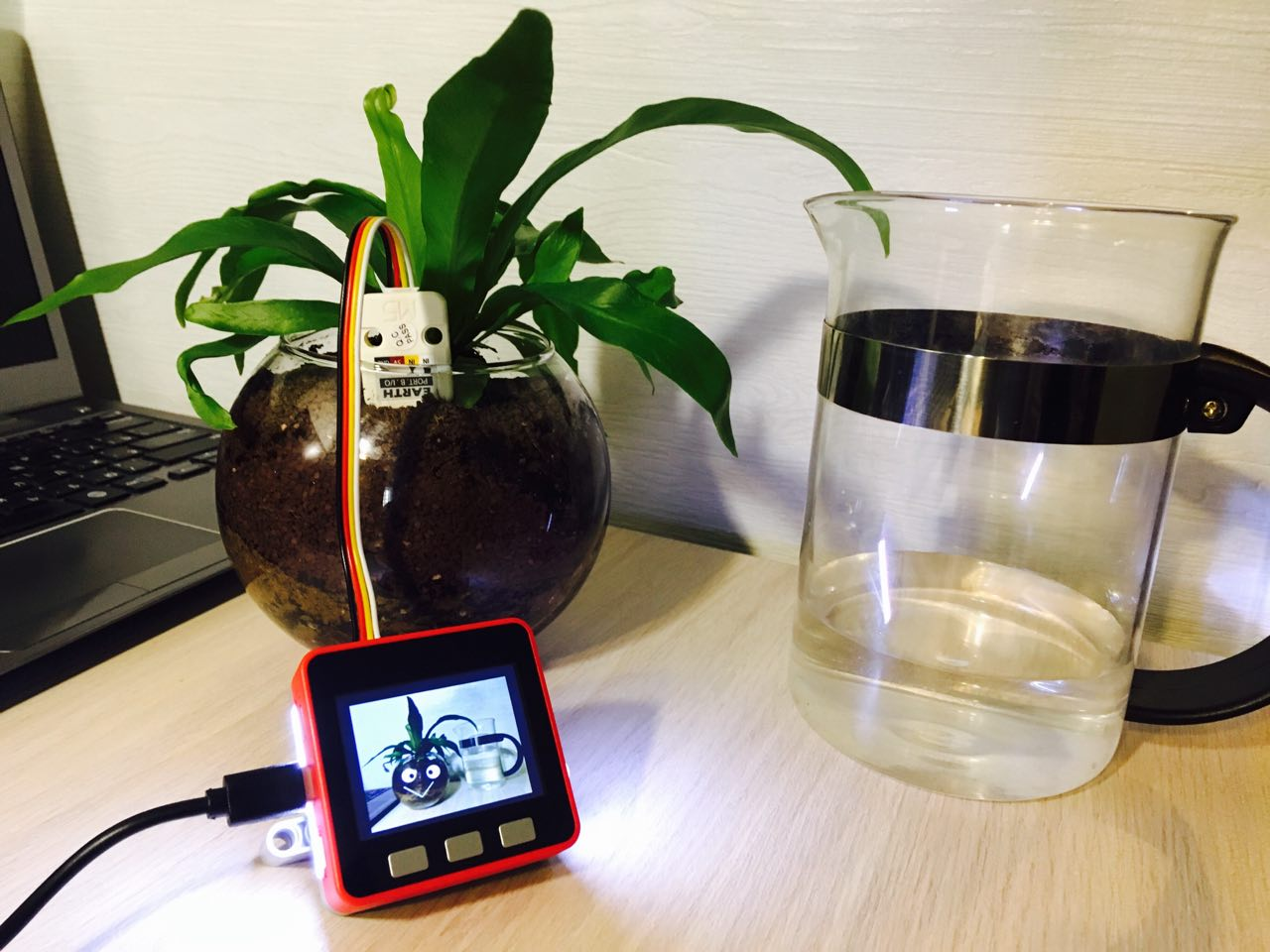Figure 1

This lesson will teach you how to obtain and process analog soil moisture data from a sensor.

Short info

• Purpose: used to determine soil moisture
• Scope: agriculture and science
• Connection interface: I / O (Groove Port b connector)
• Supply voltage: 5 V
• Compatibility: M5 FIRE
• Form factor: LEGO compatible

Brief overview

EARTH-one of the large family of additional modules (UNITS), designed primarily for M5 Fire. This module is supplied in a plastic box. The kit includes an excellent cable (15 cm) with Groove plugs (figure 2). On the front side of the sensor there is a sticker with the name of the module and the designation of contacts. Interestingly, the sensor can operate in two modes: analog and digital.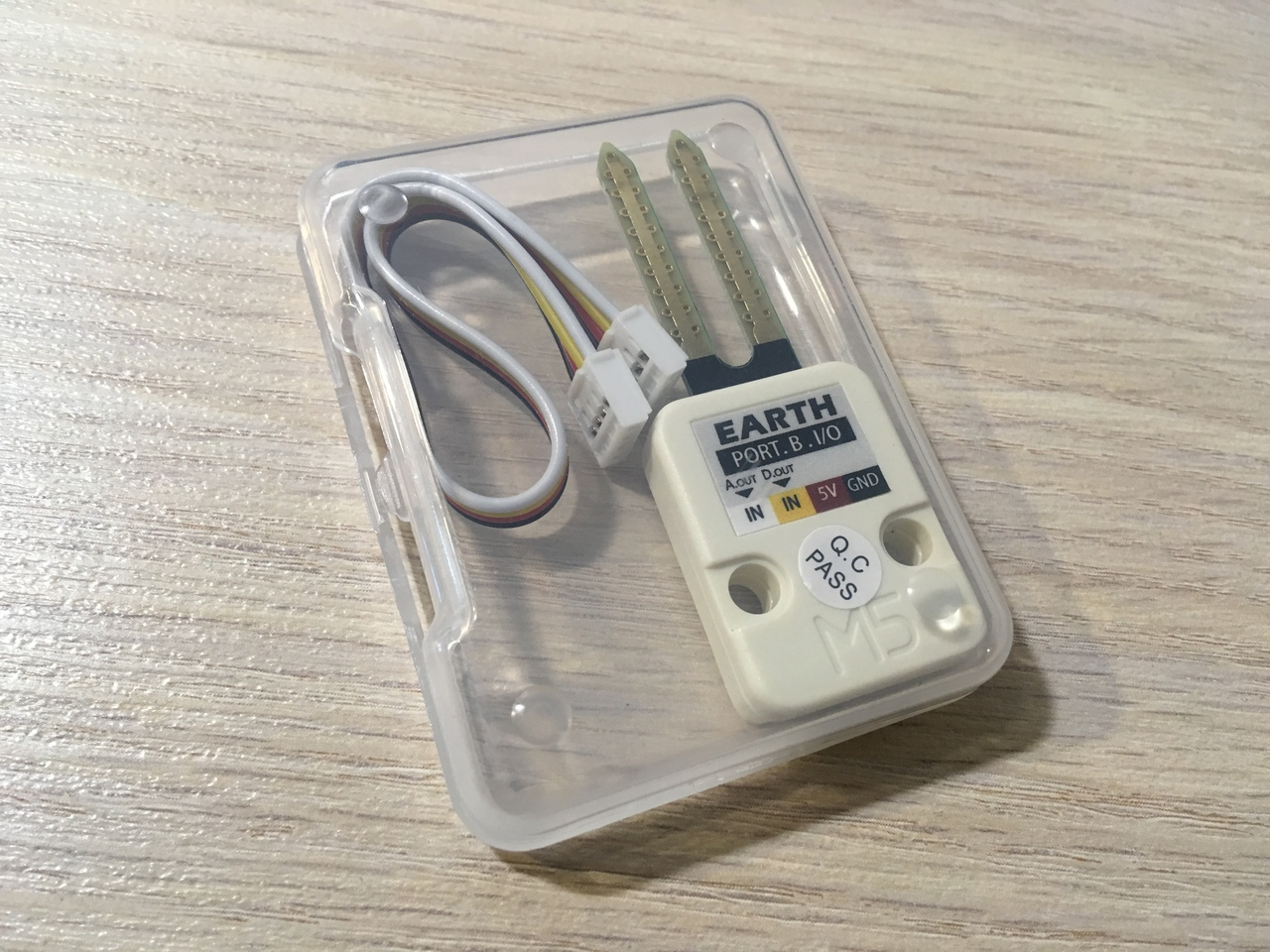Figure 2

In order to set the trigger threshold for the digital output, there is a trimmer resistor motor on the back of the sensor (figure 3), which can be rotated with a screwdriver.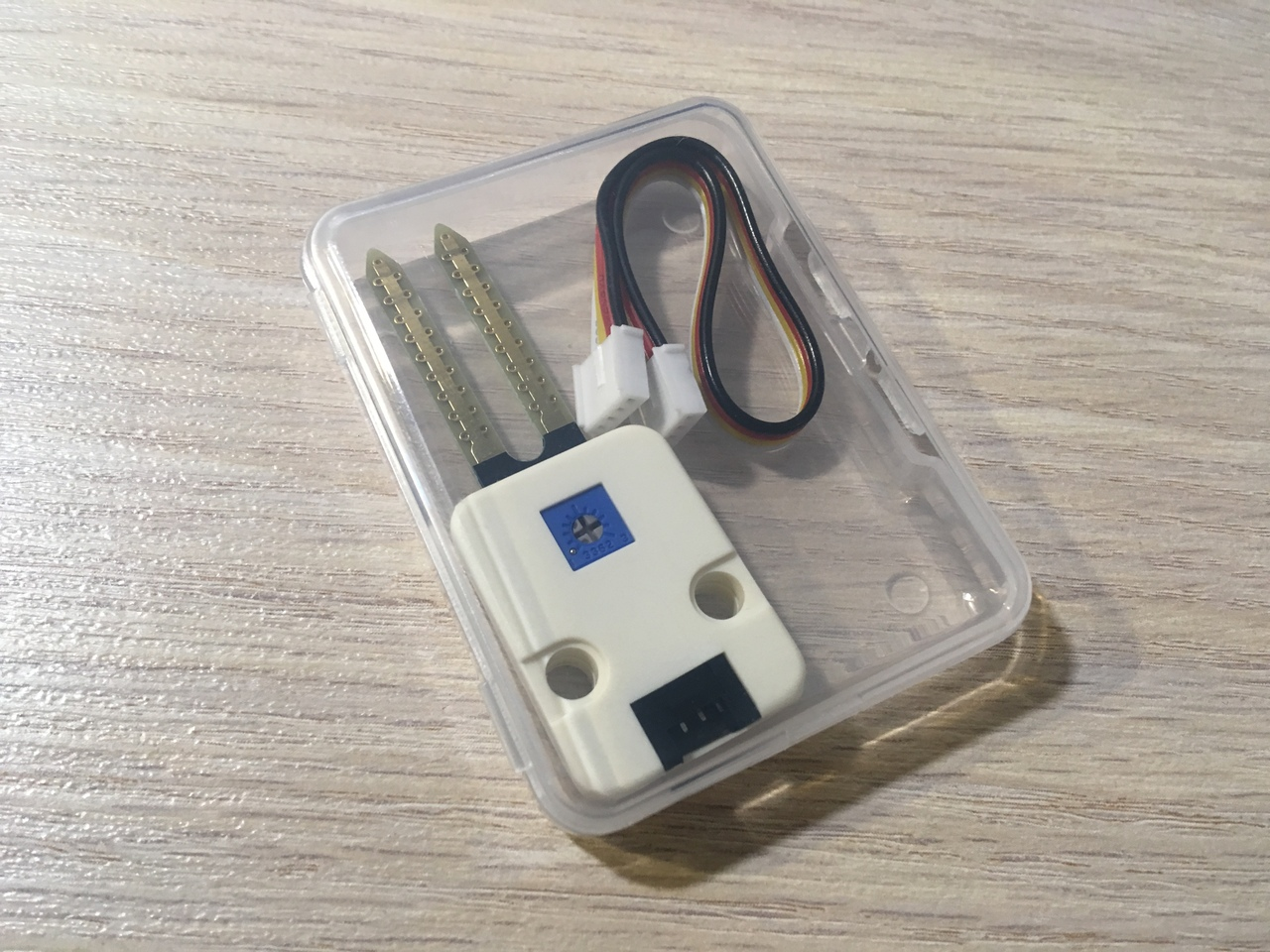Figure 3

Overall dimensions of the sensor are ridiculous, and the problems with installation is not (figure 4) :)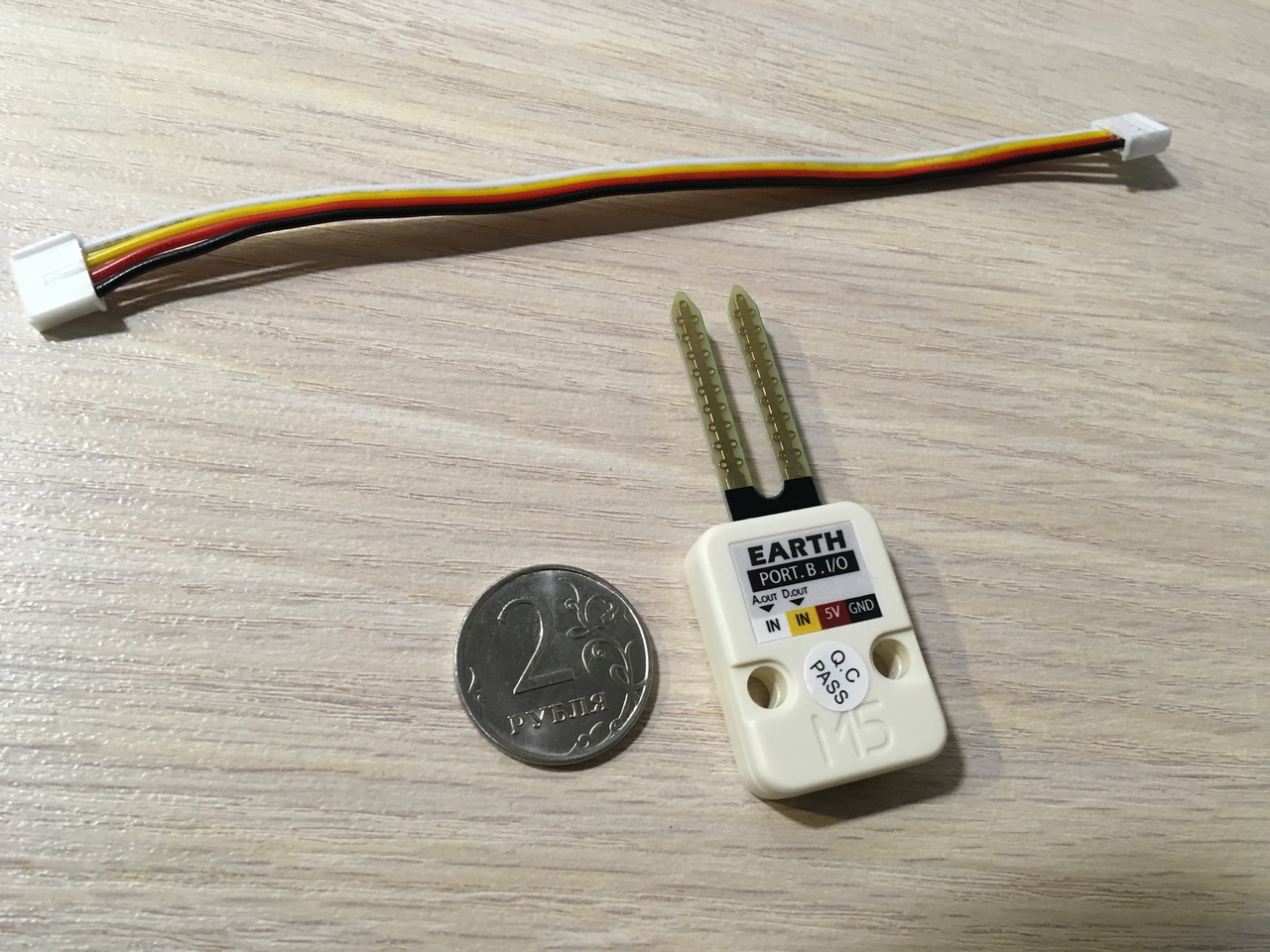Figure 4

Connect the sensor directly to the above M5 port using the supplied cable (figure 5). The sensor can be mounted with a fork in the ground to the black layer.Figure 5

Let's start!

Blockly (UI Flow)

First of all, we need to make sure that the Flow UI is connected to the M5. If You see the words "Disconneted", then repeatedly press the arrows (1) as long as the label (2) does not change to "Connected". Great! Now we can add UNITS by clicking on plus (3) (figure 6).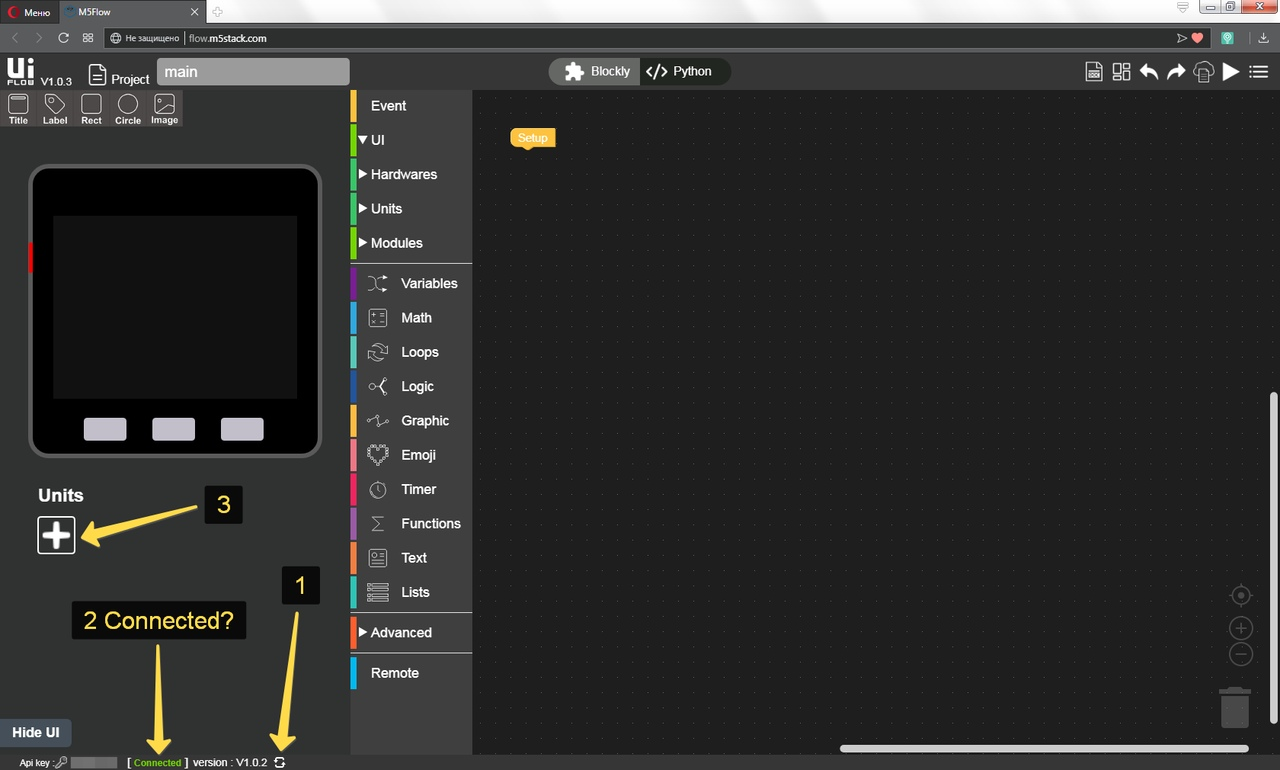Figure 6

OK, now check the box next to EARTH (1), then click OK (2) (figure 6.1).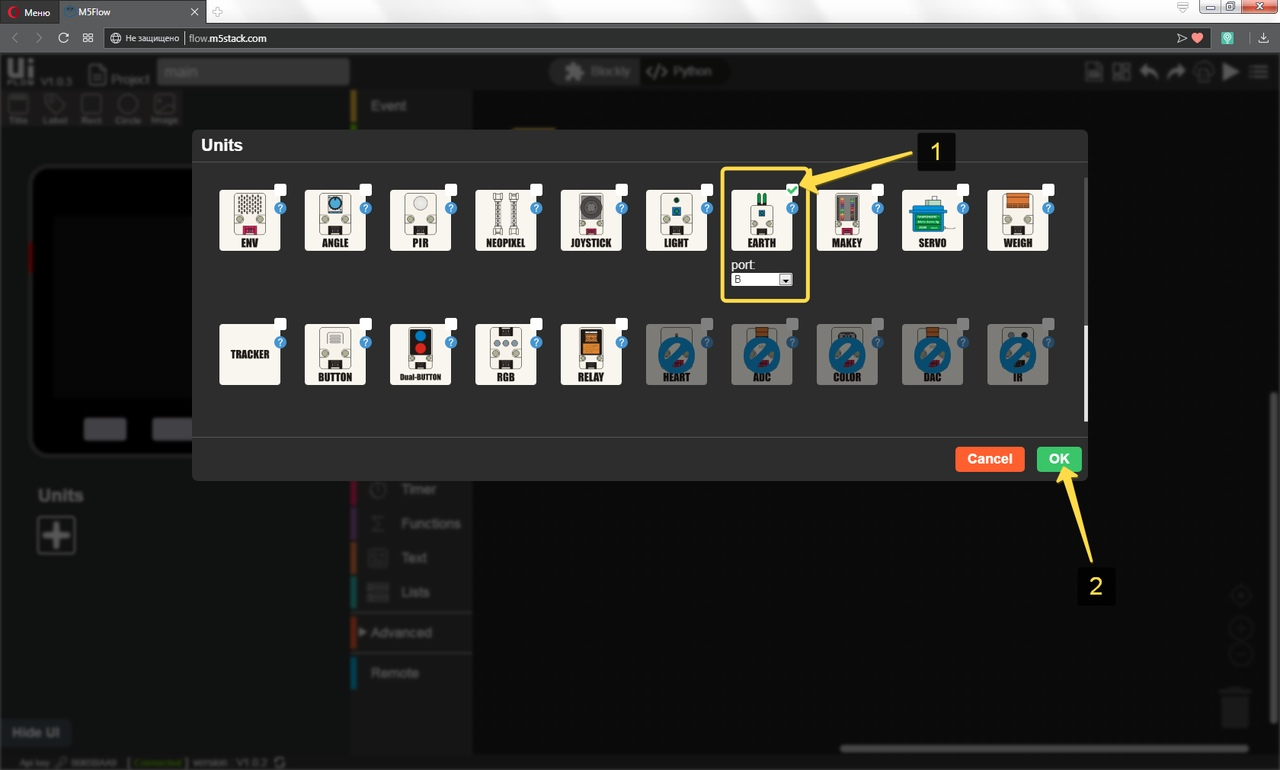Figure 6.1

In order to get analog data from the module, you need to add The corresponding blockly puzzle. Click UNITS (1), then Earth (2), then drag the Analog Value (3) puzzle to the workspace (figure 6.2).Figure 6.2

Wow! Now we want to change the image depending on the soil moisture. First, you need to download images from the Images archive.zip to the device. To do this, click on the Resource Manager icon (1) (figure 6.3).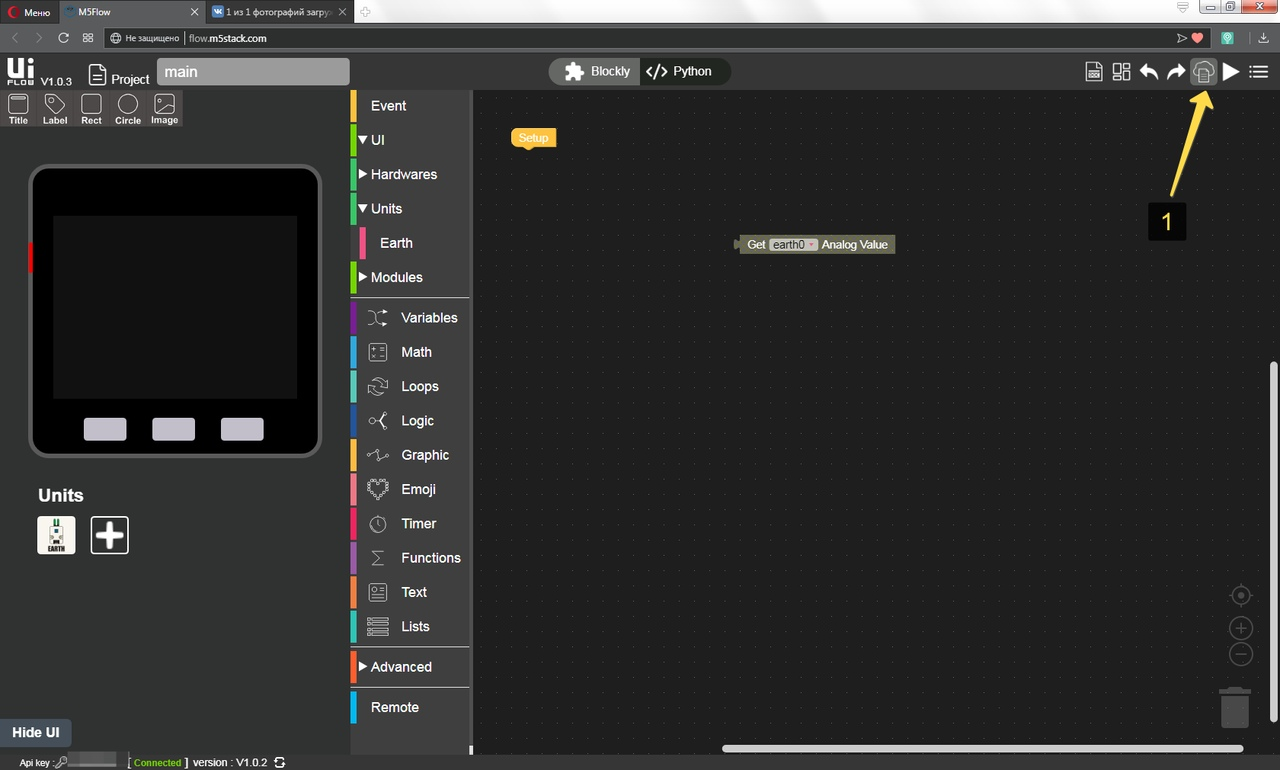Figure 6.3

Next, click the Add Image button (1) and load all four images one by one, then click Cancel (2) (figure 6.4). This completes the uploading of images to the device.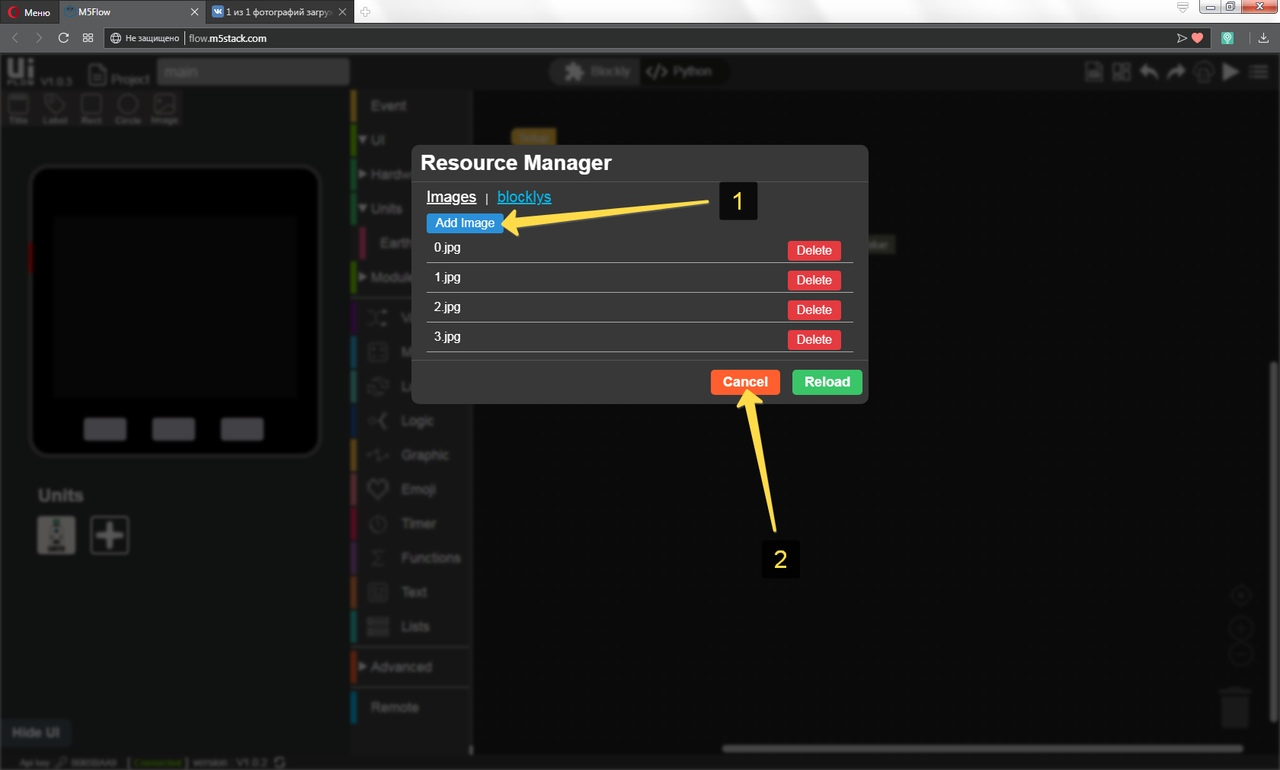Figure 6.4

Drag the Image and drop it onto the virtual device display (1). Click on the image that appears, then the properties table opens. You must change the first four properties according to the picture number (2) (figure 6.5). Repeat this step for each image.Figure 6.5

Good. Do not forget to convert the resulting value from the analog input from the float type to the integer type using the corresponding puzzle (figure 7).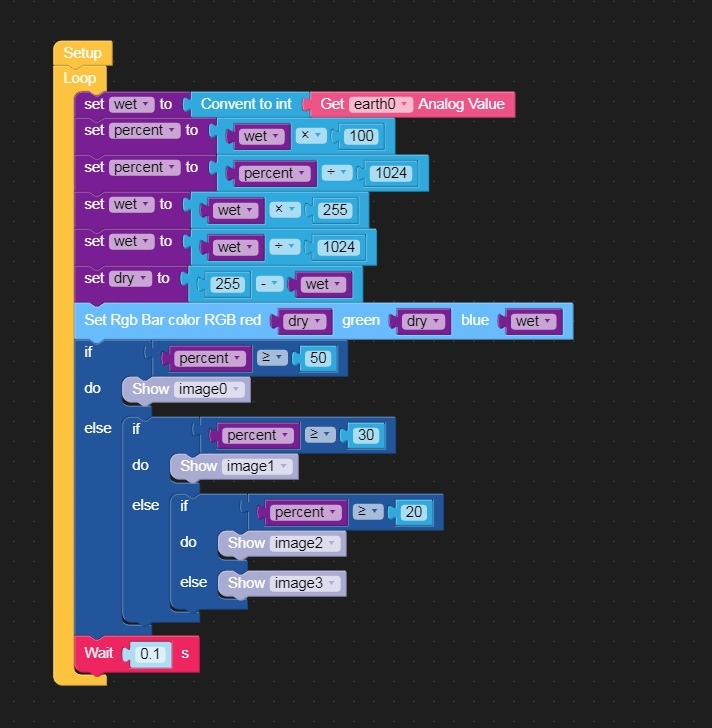Figure 7.

The lesson is finished! :)

MicroPython (UI Flow)

``````from m5stack import *
from m5ui import *
import units

clear_bg(0x111111)
earth0 = units.Earth(units.PORTB)

btnA = M5Button(name="ButtonA", text="ButtonA", visibility=False)
btnB = M5Button(name="ButtonB", text="ButtonB", visibility=False)
btnC = M5Button(name="ButtonC", text="ButtonC", visibility=False)
image0 = M5Img(20, 20, "res/0.jpg", True)
image1 = M5Img(20, 20, "res/1.jpg", True)
image2 = M5Img(20, 20, "res/2.jpg", True)
image3 = M5Img(20, 20, "res/3.jpg", True)

wet = None
percent = None
dry = None

while True:
percent = wet * 100
percent = percent / 1024
wet = wet * 255
wet = wet / 1024
dry = 255 - wet
rgb.set_all(int('0x%02x%02x%02x' % (round(min(100, max(0, dry)) * 2.55), round(min(100, max(0, dry)) * 2.55), round(min(100, max(0, wet)) * 2.55))))
if percent >= 50:
image0.show()
else:
if percent >= 30:
image1.show()
else:
if percent >= 20:
image2.show()
else:
image3.show()
wait(0.1)
wait(0.001)
``````

C & C++ (Arduino IDE)

Example not yet written ^_^